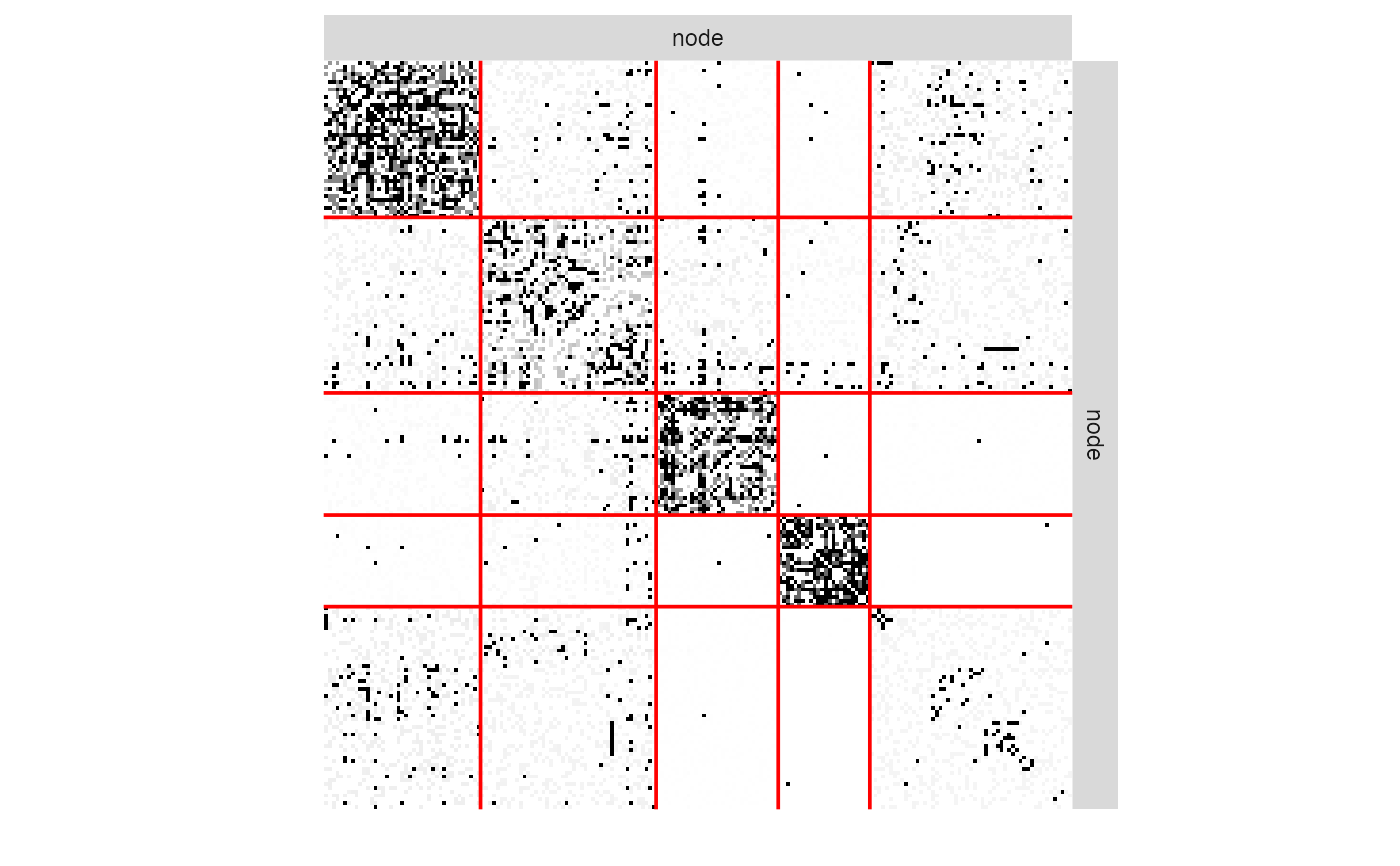The function estimateMissSBM() fits a collection of SBM for varying number of block. Each fitted SBM is an instance of an R6 object with class missSBM_fit, described here.

Fields are accessed via active binding and cannot be changed by the user.

This class comes with a set of R6 methods, some of them being useful for the user and exported as S3 methods. See the documentation for show(), print(), fitted(), predict(), plot().

## Active bindings

fittedSBM

the fitted SBM with class SimpleSBM_fit_noCov, SimpleSBM_fit_withCov or SimpleSBM_fit_MNAR inheriting from class sbm::SimpleSBM_fit

fittedSampling

the fitted sampling, inheriting from class networkSampling and corresponding fits

imputedNetwork

The network data as a matrix with NAs values imputed with the current model

monitoring

a list carrying information about the optimization process

entropyImputed

the entropy of the distribution of the imputed dyads

entropy

the entropy due to the distribution of the imputed dyads and of the clustering

vExpec

double: variational expectation of the complete log-likelihood

penalty

double, value of the penalty term in ICL

loglik

double: approximation of the log-likelihood (variational lower bound) reached

ICL

double: value of the integrated classification log-likelihood

## Methods

### Method new()

constructor for networkSampling

missSBM_fit$new(partlyObservedNet, netSampling, clusterInit, useCov = TRUE) #### Arguments partlyObservedNet An object with class partlyObservedNetwork. netSampling The sampling design for the modelling of missing data: MAR designs ("dyad", "node") and MNAR designs ("double-standard", "block-dyad", "block-node" ,"degree") clusterInit Initial clustering: a vector with size ncol(adjacencyMatrix), providing a user-defined clustering. The number of blocks is deduced from the number of levels in with clusterInit. useCov logical. If covariates are present in partlyObservedNet, should they be used for the inference or of the network sampling design, or just for the SBM inference? default is TRUE. ### Method doVEM() a method to perform inference of the current missSBM fit with variational EM #### Usage missSBM_fit$doVEM(
control = list(threshold = 0.01, maxIter = 100, fixPointIter = 3, trace = TRUE)
)

#### Arguments

control

a list of parameters controlling the variational EM algorithm. See details of function estimateMissSBM()

### Method show()

show method for missSBM_fit

### Method clone()

The objects of this class are cloneable with this method.

missSBM_fit$clone(deep = FALSE) #### Arguments deep Whether to make a deep clone. ## Examples ## Sample 75% of dyads in French political Blogosphere's network data adjMatrix <- missSBM::frenchblog2007 %>% igraph::as_adj (sparse = FALSE) %>% missSBM::observeNetwork(sampling = "dyad", parameters = 0.75) collection <- estimateMissSBM(adjMatrix, 3:5, sampling = "dyad") #> #> #> Adjusting Variational EM for Stochastic Block Model #> #> Imputation assumes a 'dyad' network-sampling process #> #> Initialization of 3 model(s). #> Performing VEM inference #> Model with 4 blocks. Model with 3 blocks. Model with 5 blocks. #> Looking for better solutions #> Pass 1 Going forward ++ Pass 1 Going backward ++ my_missSBM_fit <- collection$bestModel
class(my_missSBM_fit)
#>  "missSBM_fit" "R6"         plot(my_missSBM_fit, "imputed")